# 图像膨胀

2019/04/10 10:10

1、原理

A⊕S={z|(S)z ∩ A ≠ Ø}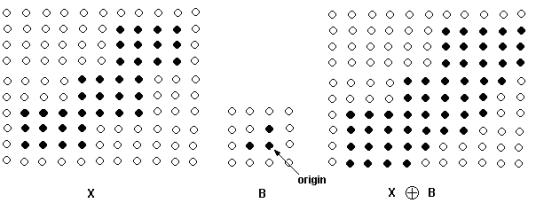2、膨胀的实现

imgPath = 'E:\opencv_pic\src_pic\pic1.bmp';
img=rgb2gray(img);
img = 255-img;

se = strel('square', 3)
img2 = imdilate(img, se);
subplot(1,2,1),imshow(img),title('原始图像');
subplot(1,2,2),imshow(img2),title('膨胀图像');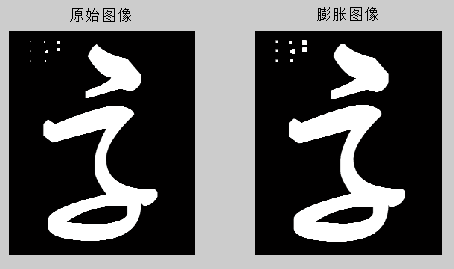放大后观察，左侧的噪点，第一列最下面一个是2*2的块，膨胀后变为4*4块。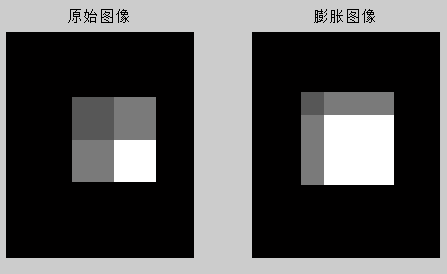se = strel('square', 5)
img2 = imdilate(img, se);
subplot(1,3,1),imshow(img),title('原始图像');
subplot(1,3,2),imshow(img2),title('膨胀图像系数5');
se3 = strel('square', 10)
img3 = imdilate(img, se3);
subplot(1,3,3),imshow(img3),title('膨胀图像系数10');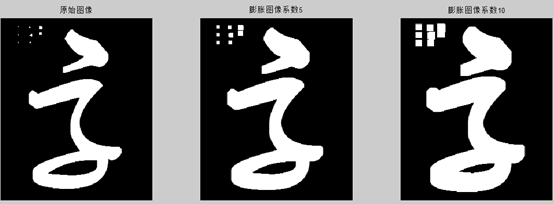imgPath = 'E:\opencv_pic\src_pic\pic1.bmp';
img=rgb2gray(img);
img = 255-img;

se = strel('square', 5)
img2 = imerode(img, se);
img3 = imdilate(img2, se);
subplot(1,3,1),imshow(img),title('原始图像');
subplot(1,3,2),imshow(img2),title('腐蚀图像');
subplot(1,3,3),imshow(img3),title('膨胀图像');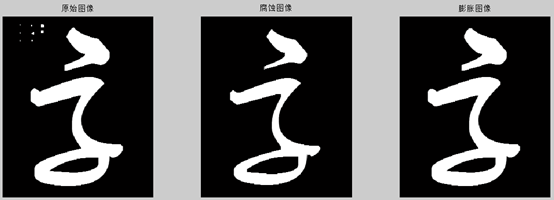3、总结

https://www.cnblogs.com/pingwen/p/12238674.html### 作者的其它热门文章

0
0 收藏

0 评论
0 收藏
0